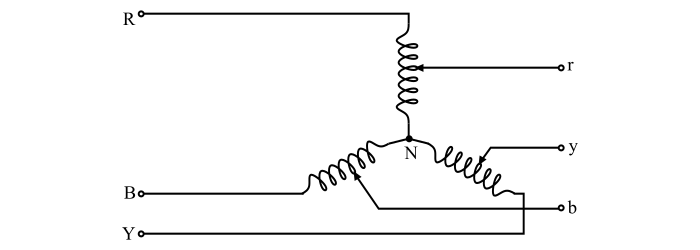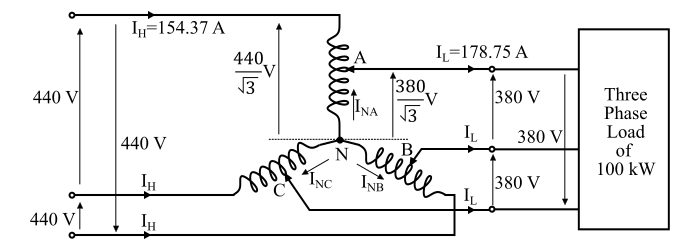# How Does a Three-Phase Autotransformer Function?

A three-phase star-connected autotransformer is shown in the figure. The three-phase autotransformers are used for small ratios of transformation.In practice, the star connected three-phase autotransformers are used and the delta connected autotransformers are avoided. The primary application of three phase autotransformers is for interconnecting two power systems of different voltage levels such as 66 kV to 132 kV power systems, 110 kV to 220 kV power systems, 220 kV to 400 kV power systems etc.

## Numerical Example

A three-phase star-connected autotransformer supplies a balanced three-phase load of 100 kW at 380 V and at 0.85 power factor lagging. If the supply voltage is 440 V, Calculate the winding currents as well as the input and output line currents. Neglect the excitation current and the internal voltage drops in the transformer.

Solution

The connection diagram of the given autotransformer is shown in the figure.The three-phase power is given by,

$$\mathrm{𝑃 = √3\:𝑉_{𝐿}\:𝐼_{𝐿}\:cos\:φ}$$

$$\mathrm{∴𝐼_{𝐿}=\frac{𝑃}{√3\:𝑉_{𝐿}\:cos\:φ}=\frac{100\:×\:10^{3}}{√3×\:380\:×\:0.85}}$$

$$\mathrm{\Rightarrow\:𝐼_{𝐿}= 178.75\:A}$$

Thus, the output line current of the given three-phase autotransformer is 178.75 A.

As the losses are neglected, therefore,

$$\mathrm{Input\:VA\:per\:phase\:(𝑉_{𝐻}𝐼_{𝐻}) = output\:VA\:per\:phase(𝑉_{𝐿}𝐼_{𝐿})}$$

$$\mathrm{\Rightarrow\:\frac{440}{√3}× 𝐼_{𝐻}=\frac{380}{√3}×\:𝐼_{𝐿}}$$

$$\mathrm{\Rightarrow\:𝐼_{𝐻}=\frac{380}{440}× 178.75 = 154.37\:A}$$

Thus, the input line current of the given three-phase autotransformer is 154.37 A.

Now, the currents flowing from neutral point (N) to tapping points A, B and C are given by applying KCL at respective tapping point, thus,

$$\mathrm{𝐼_{𝑁𝐴}= 𝐼_{𝑁𝐵}= 𝐼_{𝑁𝐶}= 𝐼_{𝐿}\:−\:𝐼_{𝐻}= 178.75\:−\:154.37 = 24.38\:A}$$

Therefore, the currents flowing in the windings of the given transformer are equal to 24.38 A.